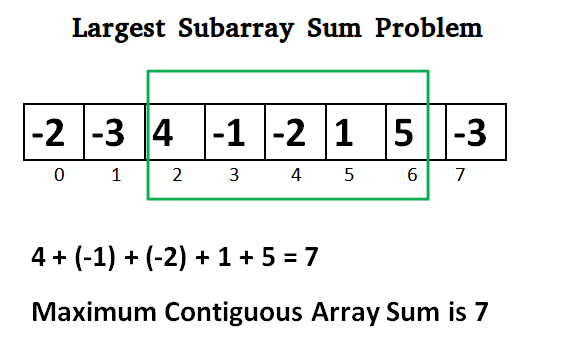Related Articles

# C / C++ Program for Largest Sum Contiguous Subarray

• Last Updated : 12 Dec, 2018

Write an efficient program to find the sum of contiguous subarray within a one-dimensional array of numbers which has the largest sum.## C++

 `// C++ program to print largest contiguous array sum``#include ``#include ``using` `namespace` `std;`` ` `int` `maxSubArraySum(``int` `a[], ``int` `size)``{``    ``int` `max_so_far = INT_MIN, max_ending_here = 0;`` ` `    ``for` `(``int` `i = 0; i < size; i++) {``        ``max_ending_here = max_ending_here + a[i];``        ``if` `(max_so_far < max_ending_here)``            ``max_so_far = max_ending_here;`` ` `        ``if` `(max_ending_here < 0)``            ``max_ending_here = 0;``    ``}``    ``return` `max_so_far;``}`` ` `/*Driver program to test maxSubArraySum*/``int` `main()``{``    ``int` `a[] = { -2, -3, 4, -1, -2, 1, 5, -3 };``    ``int` `n = ``sizeof``(a) / ``sizeof``(a);``    ``int` `max_sum = maxSubArraySum(a, n);``    ``cout << ``"Maximum contiguous sum is "` `<< max_sum;``    ``return` `0;``}`
Output:
```Maximum contiguous sum is 7
```

Above program can be optimized further, if we compare max_so_far with max_ending_here only if max_ending_here is greater than 0.

## C++

 `int` `maxSubArraySum(``int` `a[], ``int` `size)``{``    ``int` `max_so_far = 0, max_ending_here = 0;``    ``for` `(``int` `i = 0; i < size; i++) {``        ``max_ending_here = max_ending_here + a[i];``        ``if` `(max_ending_here < 0)``            ``max_ending_here = 0;`` ` `        ``/* Do not compare for all elements. Compare only   ``          ``when  max_ending_here > 0 */``        ``else` `if` `(max_so_far < max_ending_here)``            ``max_so_far = max_ending_here;``    ``}``    ``return` `max_so_far;``}`

Please refer complete article on Largest Sum Contiguous Subarray for more details!

Want to learn from the best curated videos and practice problems, check out the C Foundation Course for Basic to Advanced C.

My Personal Notes arrow_drop_up